### IMO Shortlist 2011 problem G5

Kvaliteta:
Avg: 0,0
Težina:
Avg: 8,0
Let$ABC$ be a triangle with incentre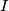$I$ and circumcircle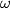$\omega$. Let$D$ and$E$ be the second intersection points of$\omega$ with$AI$ and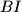$BI$, respectively. The chord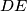$DE$ meets$AC$ at a point$F$, and$BC$ at a point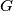$G$. Let$P$ be the intersection point of the line through$F$ parallel to$AD$ and the line through$G$ parallel to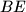$BE$. Suppose that the tangents to$\omega$ at$A$ and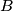$B$ meet at a point$K$. Prove that the three lines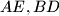$AE,BD$ and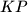$KP$ are either parallel or concurrent.

Proposed by Irena Majcen and Kris Stopar, Slovenia
Izvor: Međunarodna matematička olimpijada, shortlist 2011